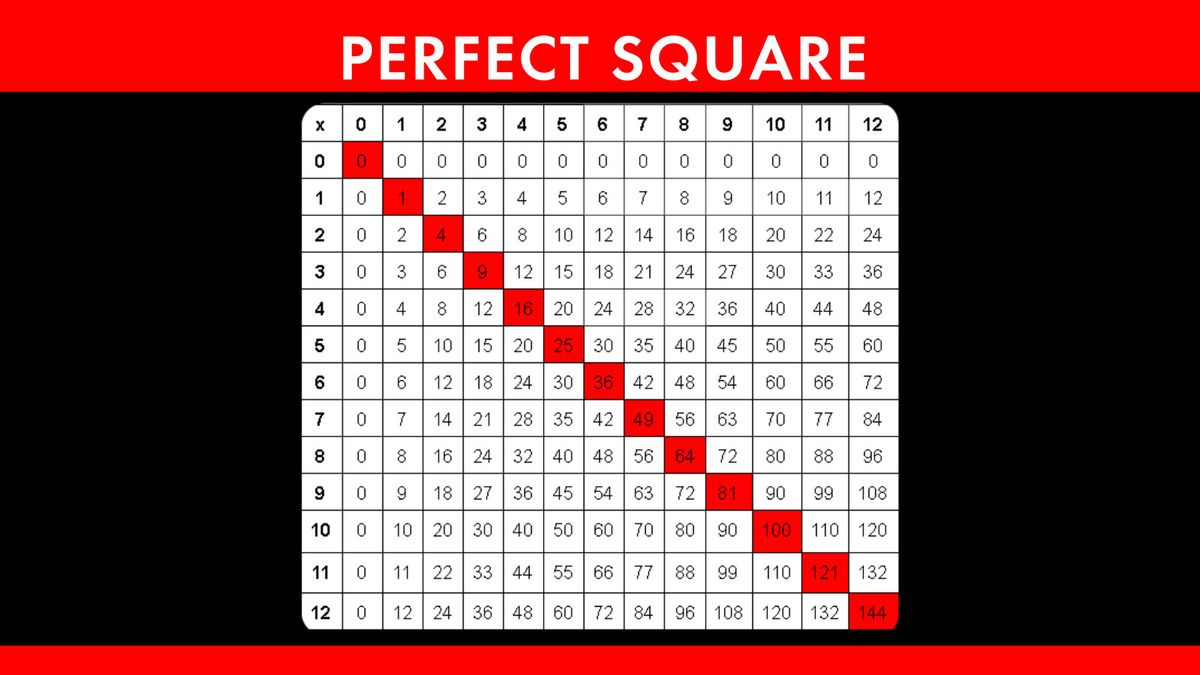# What Is a Perfect Square?So, how can you determine if a number is a perfect square? The trick lies in finding its square root, which is the reverse operation of squaring a number. If the square root yields a whole number, you’re looking at a perfect square.

For instance, the square root of 16 is 4 because 4 x 4 = 16. Therefore, 16 is indeed a perfect square. Likewise, if you calculate the square root of 144, you’ll find it’s 12 because 12 x 12 = 144. Bingo! Another perfect square.

### Perfect Squares Chart

To help you get a better grasp of perfect squares, here are a few more common examples:

• 1 x 1 = 1
• 2 x 2 = 4
• 3 x 3 = 9
• 4 x 4 = 16
• 5 x 5 = 25
• 6 x 6 = 36
• 7 x 7 = 49
• 8 x 8 = 64
• 9 x 9 = 81
• 10 x 10 = 100

As you can see, each of these numbers can be expressed as the product of two equal integers, making them perfect squares.